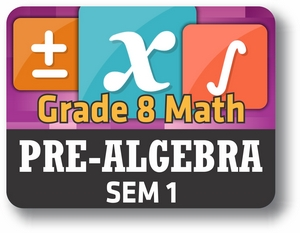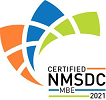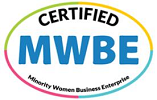Red Comet## Course Details:

This course covers all the important topics necessary before a student takes on a full-fledged Algebra course. Student learns about the different types of numbers, their properties and the different operations that can be performed on them. They also learn about ratios & proportions, rates and percent. The course also teaches about measurement and conversions.

## Syllabus:

Section 1: Numbers
• Rational Numbers
• Compare Rational Numbers
• Absolute Value and Opposites
• Number Line
• Converting Fractions to Decimals and Decimals to Fractions
• Classify Numbers
• Square Roots
• Cube Roots
Section 2: Operations
• Basic operations on Integers
• Order of Operations (Integers)
• Variable Expressions (Integers)
• Add and Subtract Rational Numbers
• Multiply and Divide Rational Numbers
• Order of Operations
• Variable Expressions (Rational Numbers)
• Word Problems
Section 3: Ratios, Rates and Proportions
1. Ratios and Proportions
2. Equivalent Ratios
3. Unit Rates
4. Unit Prices
5. Solve Proportions
6. Scale
7. Word Problems
Section 4: Percent
• Introduction to Percent
• Converting Percentages
• Percent Change
• Percent Equation
• Percent Applications
• Word Problems
Section 5: Measurements
• Customary Units
• Metric Units
• Unit Prices
• Precision
• Greatest Possible Error
• Minimum and Maximum in Area and Volume
• Percent Error
• Percent Error in Area and Volume
• Word Problems

### Accreditation & Approvals